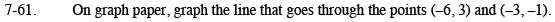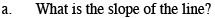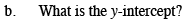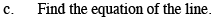Home > CAAC > Chapter 7 > Lesson 7.2.1 > Problem7-61

7-61.
1. On graph paper, graph the line that goes through the points (−6, 3) and (−3, −1). 7-61 HW eTool (Desmos). Homework Help ✎

1. What is the slope of the line?

2. What is the y-intercept?

3. Find the equation of the line.Draw a slope triangle to calculate the rise over the run.

$-\frac{4}{3}$At what point does the line cross the y-axis?

(0, −5)Remember y = mx + b.

Use the table in the eTool below to graph the line.
Click the link at right for the full version of the eTool: AC 7-61 HW eTool